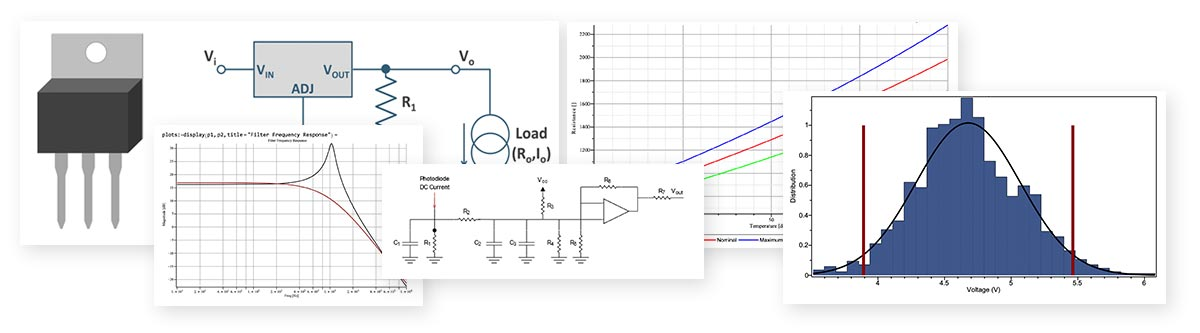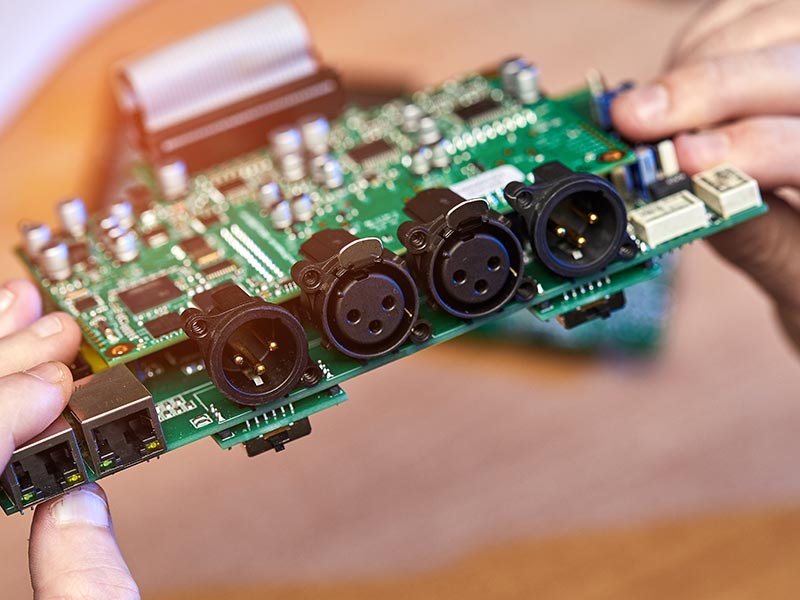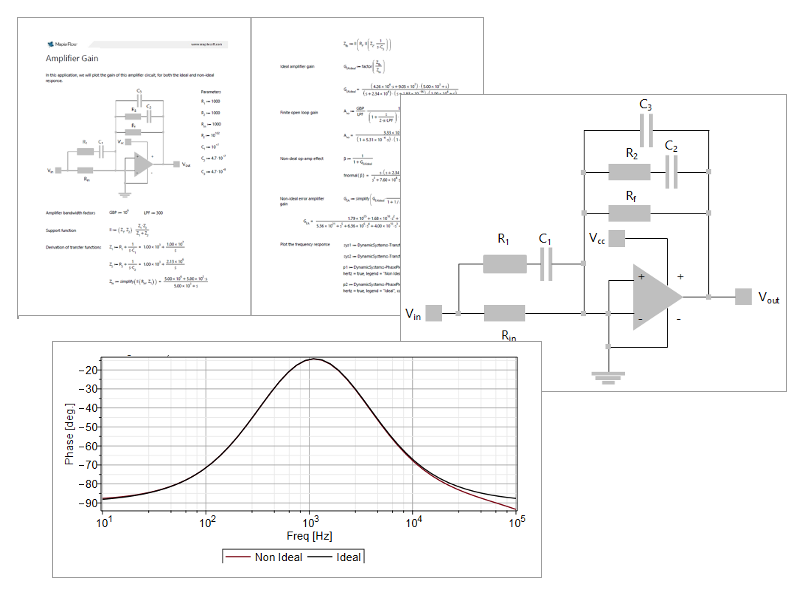Worst-Case Circuit Analysis – Maple Flow

# Mathematical Tools for Worst-Case Circuit Analysis

• Derive circuit equations
• Analyze circuit tolerances with WCCA, EVA, RSS, Monte-Carlo simulation and sensitivity analysis
• Generate design reportsUsing Worst-Case Circuit Analysis
Worst-Case Circuit Analysis (WCCA) is a set of techniques used to determine electronic circuit performance under extremes of environmental or operating conditions. WCCA shows how parameter variations combine to influence circuit performance, and helps the engineer shape the design so the overall system will remain compliant throughout its life-cycle.

Using Maple Flow to manage your worst-case analysis projects, you can ensure that the circuit satisfies the performance requirements over the full range of component parameters and unfavourable operating conditions.
• Use Maple Flow if you need a mathematical scratchpad for efficiently implementing WCCA
• Use Maple if you want greater control over report generation and programmatically generated tables of results

# Are You a Mathcad® User?

Special, competitive upgrade pricing is available for Mathcad users switching to Maple Flow.

Do you want to work on mathematically-focused problems, solve larger systems, and develop algorithms? Check out Maple

Maple Flow provides a freeform, paper-like, calculation environment.

• Do calculations in readable, natural math notation, with full support for units, and easy-to-use solvers
• Create immersive, engaging design reports with text, equations, images and plots
• Generate attractive plots and chartsMaple Flow lets you do Worst-Case circuit analysis of components in electric circuits. You can implement these techniques:
• Extreme value analysis (EVA)
• The behaviour of a circuit is simulated for every permutation of extreme component parameters. E.g., a resistor of 5 Ω ± 5% is simulated at 4.75 Ω  and 5.25 Ω, in combination with every permutation of extreme values for all other components.
• Both symmetric and asymmetric tolerances can be implemented.
• Monte Carlo Analysis (MCA)
• Parameters are randomly selected from a distribution, and the circuit simulated, anywhere from 1000 to 100000 times
• You can generate histograms, calculate the minimum and maximum values, view the statistical distribution of the results and more.
• Sensitivity analysis (SA)
• You can calculate the symbolic or numeric partial derivatives of the circuit with respect to each component parameter. These can be used to perturb the circuit equations
• You can analyse the frequency-dependent behaviour with phase and magnitude plots.
• Root-sum-square (RSS) analysis
• This uses a statistical approach, assuming that most of the components fall to the mid of the tolerance zone rather than at the extremes.
• Optimization
• You can optimize the circuit equations by varying the component values within a specific range.

You can also symbolically derive circuit equations, by applying Kirchoff’s current and voltage laws. The resulting equations can be symbolically rearranged and simplified. If the equations are transfer functions, you can generate phase and magnitude plots from the transfer functions.

Given the results, circuits can be redesigned to minimize failures due to parameter variations (or an initially overdesigned circuit could be made cheaper to manufacture with less costly components that have a broader parameter distribution).
See how Maple Flow can improve your design worksheets.

###Applications for Worst-Case Circuit Analysis

Discover how Electrical Engineers use Maple Flow and Maple for the tolerance analysis of electrical circuits.

## Improve reliability across unfavourable operating conditions

Use of WCCA aims to assure acceptable operation under the most extreme conditions and during improper application. Prior to procurement of parts, engineers can conduct Extreme Value Analysis (EVA) to determine the mathematical sensitivity of component parameters and document the min/max values in Maple Flow. This avoids the over-reliance on testing alone, which is only valid for a particular batch lot and can overstress the hardware when exposing components to extreme conditions such as temperature, voltage, power, etc.## Understand the parts with the biggest impact

Some components will have a greater influence over the circuit functional performance than others. Power supplies, connectors and interfaces are expected to vary outputs even in normal operation, but every component has a contributing effect. Sensitivity Analysis (SA) is used to find how much a particular circuit characteristic varies as the component input values change. The results shape design specifications of the circuit and can be used to highlight which parts should be prioritized for quality assurance or for additional testing.## Control fluctuations in the manufacturing process

Electrical components (such as resistors and capacitors) are manufactured in large quantities. Inconsistencies in raw materials or processing quality can affect component performance. Given the number of components in a circuit and the distribution of their parameters, the circuit may not perform as specified. This risk must be identified, managed and mitigated early in the design process.
The performance fluctuation may have a statistical distribution (e.g., the resistance of a batch of resistors might be described by a normal distribution). Engineers can use the computational power of Maple Flow to perform Root-Sum-Square (RSS) or Monte Carlo Analysis to evaluate the part tolerances and pin down the margins within the circuit design.## Identify design concerns and performance thresholds

Maple Flow is the ideal tool for compiling the circuit design considerations. Equations and plots can be added alongside design notes using the flexible paper-like worksheet.  Prominent design sections such as safety thresholds, material performance properties, and assumptions about aging and environmental conditions can be clearly presented to create a polished, professional technical document.